# Dilution factor

in StemSocial6 months ago
Greetings dear friends of Hive.

In a chemistry laboratory the preparation of solutions is one of the basic and fundamental procedures to carry out any experiment. Basically a whole procedure can end badly if from the beginning the solutions with which you are going to work are badly prepared. And especially dilutions are very important, that is, the preparation of a liquid solution starting from one that has a higher concentration, adding more solvent.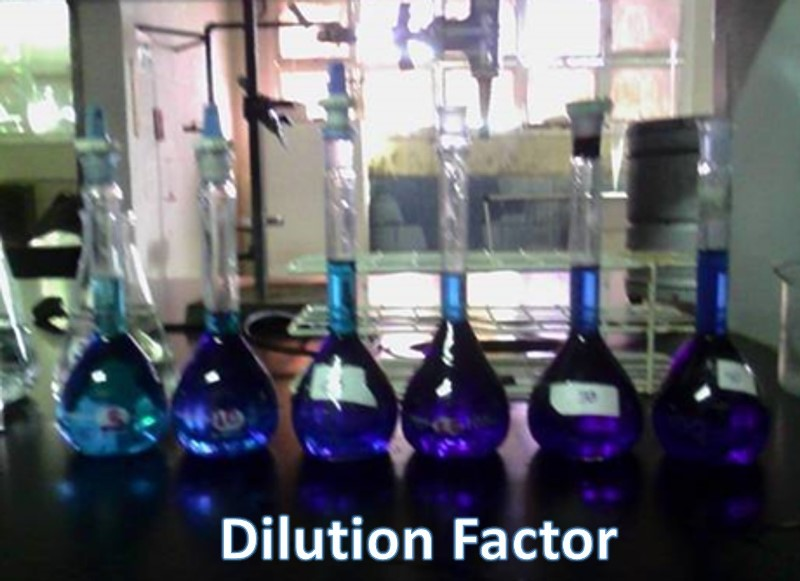Source: @emiliomoron.

Dilutions are very common since, for example, the acids that come to the laboratory are supplied in high concentrations, but in most cases they are required to be used at very low concentrations, so solutions called stock solutions are prepared from which the necessary dilutions are made to obtain the required concentrations, or sometimes we need to prepare a very diluted solution, so it is easier to make successive dilutions to avoid measurement errors that can be introduced by trying to take a very small volume of the stock solution, that is why we need to know how to determine the dilution factor.

## What does this factor consist of?

As we have already mentioned, dilution is the act of reducing the concentration of a solution by adding more solvent, but to successfully dilute a solution it is not enough to add more solvent, we must know how many times the concentration of the diluted solution must be lower with respect to the stock solution.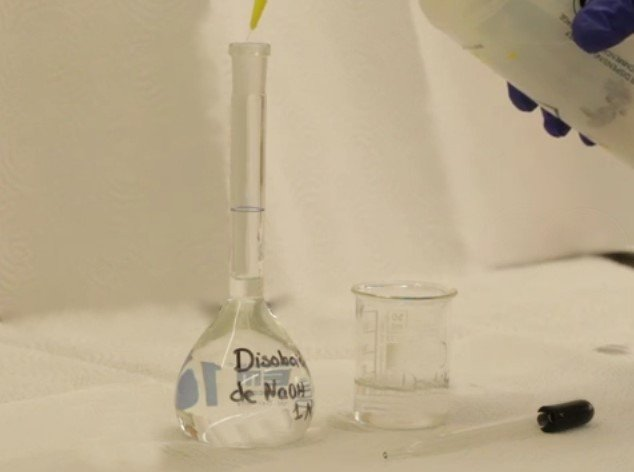Dilution of a NaOH solution. Source: @emiliomoron.

Thus, the dilution factor is a fraction that expresses how many times a concentrated solution must be diluted to obtain a solution of lower concentration. This factor corresponds to the ratio between the volume of the prepared (diluted) solution and the volume of the original (concentrated) solution.

And because the amount of solute does not vary during dilution, it can also be considered as the ratio of the concentration of the original solution to the concentration of the diluted solution, which also allows the expression to be valid for any unit of chemical concentration.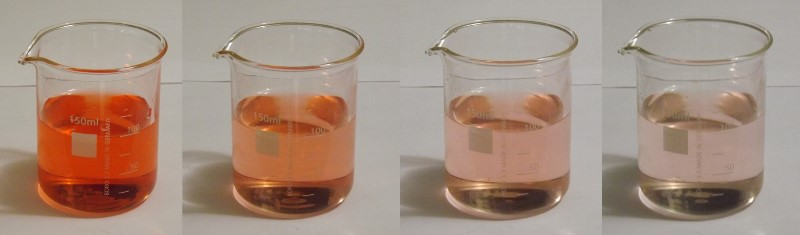Successive dilution of a sample. Source: @emiliomoron.

The image above shows an example of a successive dilution of a vegetable dye sample. In each case 50 mL of each solution has been taken and diluted again to 100 mL, with which we can observe that the color of the dilution becomes lighter, significant of a lower concentration.

But to speak of diluted and concentrated is something relative, for precise calculations we need to be able to establish a relation between the magnitudes, thus, by means of the dilution factor we can estimate how diluted is the solution of the last glass with respect to the first one. In this way, we proceed in laboratories with any reagent; concentrated stock solutions are prepared from which aliquots are taken and diluted in a certain volume necessary to give a lower concentration, thus saving reagents and also reducing the error due to pipetting very small volumes of solution.

### How to use the factor

In the laboratory we may often find that we have to prepare a 1/10 or 1/100 dilution. This tells us that to obtain the required concentration solution we must dilute the stock solution as many times as the denominator expresses, i.e. take a known volume of the stock solution (Vi) and add enough solvent to it so that the final solution has a new volume of 10 or 100 times the initial volume (Vf).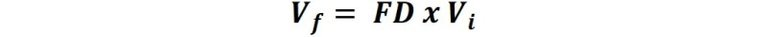The 1/10 or 1/100 represents the ratio of volumes to be used to prepare the new solution. If we consider the 1/10 as an example, it does not say to dilute the initial solution 10 times, therefore, 10 is the dilution factor (DF).

A dilution factor will not tell you what the initial volume is, nor will it tell you what the final volume is, it will only tell you what the ratio of the initial volume to the final volume is.

### Deduction of the dilution factor

Suppose that we are going to prepare a solution starting from one of higher concentration, what we do is to take a certain volume and transfer it to a volumetric balloon to add distilled water up to the mark of the volumetric gauge. In this case, the initial moles of the solute before and after adding water is the same: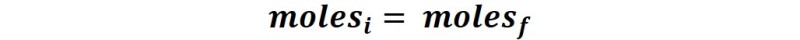From the definition of molarity (M) we can express that:Where Mi is the molar concentration of the stock solution, Vi is the volume of the aliquot taken to prepare the solution, Mf is the concentration of the solution to be obtained and Vf is the volume of the diluted solution to be prepared.

This equation can be rewritten as:The ratio Mi/ Mf tells us how many times the concentration of the stock solution is higher than the diluted one, which by definition is the dilution factor, but in the same way, it can be concluded that the ratio Vi/Vf expresses the same information.

## Calculation examples

How would 250 mL of a 0.1 M glucose solution be prepared from a 1 M solution, what is the dilution factor used?

The unknown is how much starting solution to use, then:To prepare this solution, 25 mL of the stock solution must be taken, transferred to the volumetric balloon and completed with water up to 250 mL. Therefore,The dilution factor is 1/10.

Well friends, dilution is a routine operation in any laboratory, so knowing the dilution factor is of great importance especially when there are methods and laboratory equipment that ask to make a dilution or a series of dilutions to build a calibration curve.

I hope this information will help you to learn a little more about the common procedures in a chemistry laboratory. See you next time!

## References

1. Wikipedia.com. Dilución en serie.
2. Ausetute.com. Dilution factor.
Sort:Thanks for your contribution to the STEMsocial community. Feel free to join us on discord to get to know the rest of us!

Please consider supporting our funding proposal, approving our witness (@stem.witness) or delegating to the @stemsocial account (for some ROI).

Please consider using the STEMsocial app app and including @stemsocial as a beneficiary to get a stronger support.

Thank you @emiliomoron for sharing these lines. Knowledge strengthens, refreshes, and comes loaded with experiences. Happy start of the week.